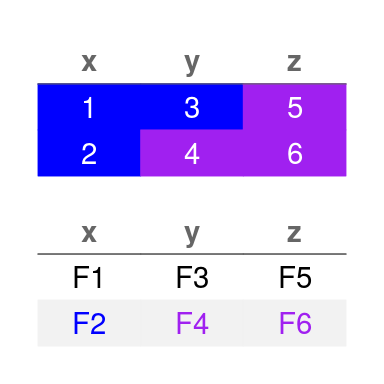# Controlling Matrix Aesthetics

#### 2020-11-29

• One of the most aesthetically customizable aspects of grobblR is the cell-by-cell aesthetics of data frames / matrices.
• The grob_matrix() function, along with add_aesthetic(), add_structure() and alter_at(), help with just this, attempting to make the process as smooth and intuitive as possible.
• The entire grob_matrix() process is intended to be pipe-friendly.
library(grobblR)

## Matrix Groups

• There are 3 types of groups the user can alter within a grob matrix:

• cells
• column_names
• column_headings
• All matrices / data frames inputted will have cells but they don’t have to have column_names, and they won’t have column_headings until the user adds them in after grob_matrix().

## grob_matrix()

• To initialize the aesthetic / structure manipulation process, the user must apply grob_matrix() to his/her matrix/data frame.
• Using view_grob() the user can view the current state of the grob matrix before inserting it into a grob-layout and producing the final PDF report.

df = data.frame(x = c(1, 2), y = c(3, 4), z = c(5, 6))

df %>%
grob_matrix() %>%
view_grob()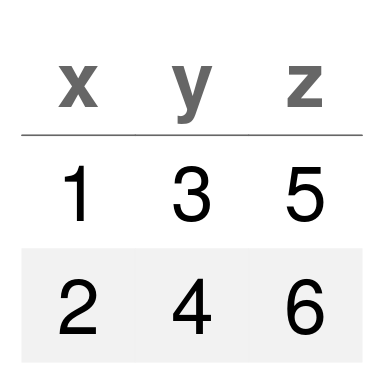• Aesthetics will be defined as any aspects of the matrix that affect its visual appearance / looks, like colors or font faces.
• add_aesthetic() applies a specific aesthetic to the entire group of the matrix.
• To see a full list of accepted matrix aesthetics, run ?add_aesthetic.

df %>%
grob_matrix() %>%
aesthetic = "text_color",
value = "red",
group = "cells"
) %>%
view_grob()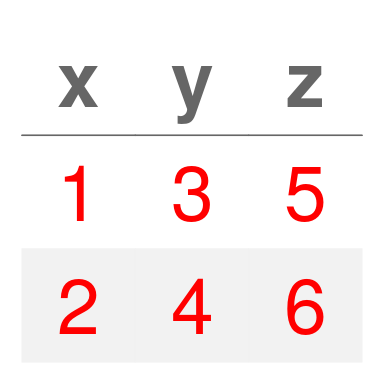df %>%
grob_matrix() %>%
aesthetic = "text_color",
value = "red",
group = "cells"
) %>%
aesthetic = "text_color",
value = "blue",
group = "column_names"
) %>%
view_grob()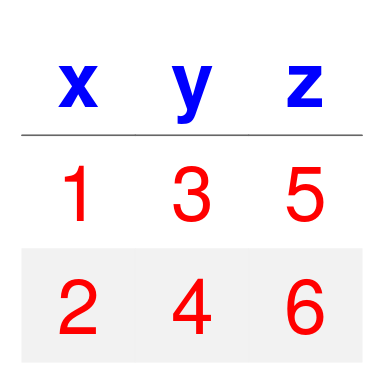• Structures will be defined as any aspects of the matrix that affect its shape, like padding or column widths.
• add_structure() applies a specific structure to the entire matrix, and not just a specific group of the matrix like adding an aesthetic.
• To see a full list of accepted matrix structures, run ?add_structure.
• The most applicable structure to matrices is column_widths_p, where providing width proportions for each of the columns is the most useful.

df %>%
grob_matrix() %>%
structure = "column_widths_p",
value = c(3, 1, 1)
) %>%
view_grob()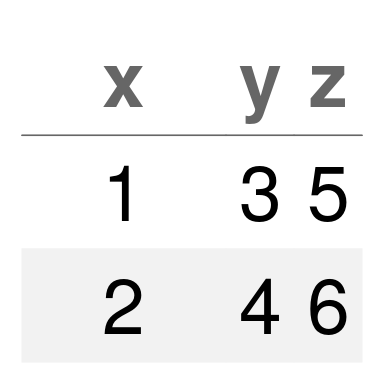• Giving a width proportion of 3 to the first column and a width proportion of 1 to the other two means x will be given 3 times the amount of width as y and z.
• Default width proportions are determined by the width of the elements in each column.

• To add column headings to a grob matrix add_column_headings() must be utilized after grob_matrix().

df %>%
grob_matrix() %>%
) %>%
view_grob()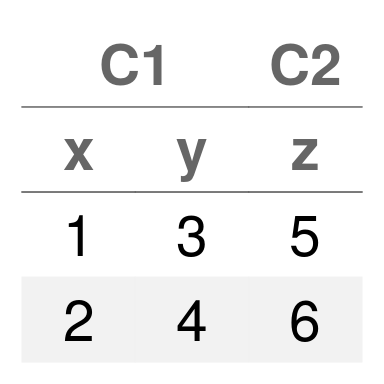df %>%
grob_matrix() %>%
) %>%
) %>%
view_grob()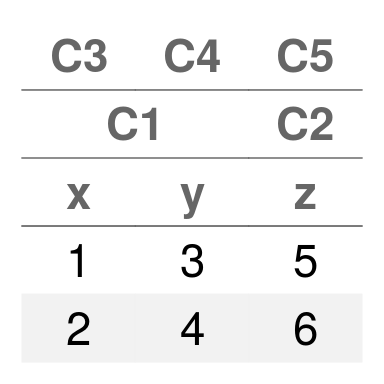• If a heading_cols is omitted, it will be filled with an empty space.

df %>%
grob_matrix() %>%
) %>%
view_grob()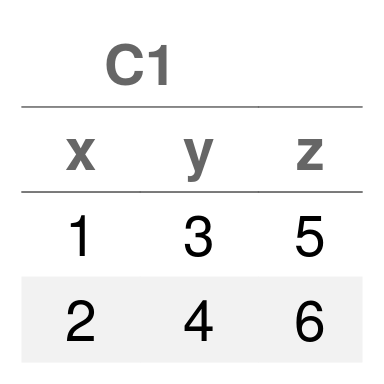• Once column headings have been initialized, the user can manipulate its aesthetics.

df %>%
grob_matrix() %>%
) %>%
aesthetic = "background_color",
value = "blue",
) %>%
aesthetic = "text_color",
value = "white",
) %>%
view_grob()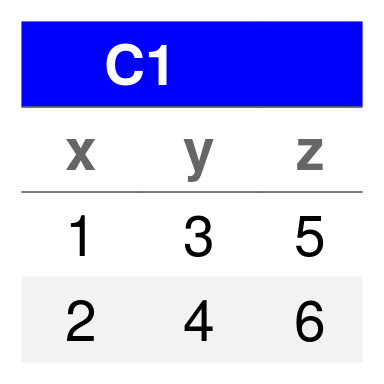## alter_column_names()

• If the user wants to change the displayed column names to something other than the initial column names passed through grob_matrix(), the user should utilize alter_column_names().

df %>%
grob_matrix() %>%
alter_column_names(
column_names = list("C1", "C2", "C3"),
column_name_cols = list(1, 2, 3)
) %>%
view_grob()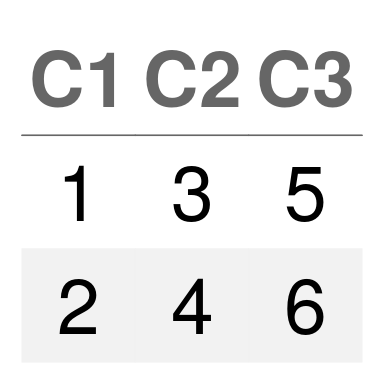• Very useful for creating column names that span across multiple columns.

df %>%
grob_matrix() %>%
alter_column_names(
column_names = list("GROUP"),
column_name_cols = list(1:2)
) %>%
view_grob()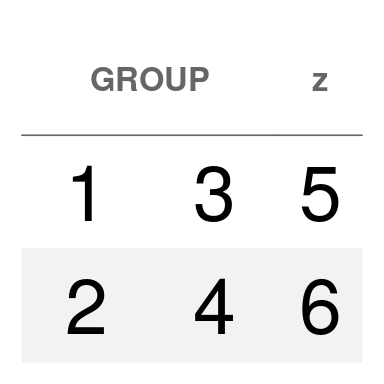## alter_at()

• alter_at() is the function that is truly empowers the user to implement cell-by-cell aesthetic / structure customization.
• Inherits the aesthetic / structure and group from the most previous add_aesthetic() / add_structure() / alter_at().
• Otherwise, the user must specify the specific aesthetic and group he/she wants to alter.
• The user can select specific columns and/or rows to apply a value/function to.
• Selected rows must be numeric row indices.
• Selected columns can either be column names or column indices.
• If no columns/rows are specified then all columns/rows will be altered.
• The value, or the .f argument of alter_at() must be a quosure style lambda ~ fun(.).

df %>%
grob_matrix() %>%
aesthetic = "text_color",
value = "blue",
group = "cells"
) %>%
alter_at(
~ "red",
columns = c("x", "y"),
rows = 1
) %>%
view_grob()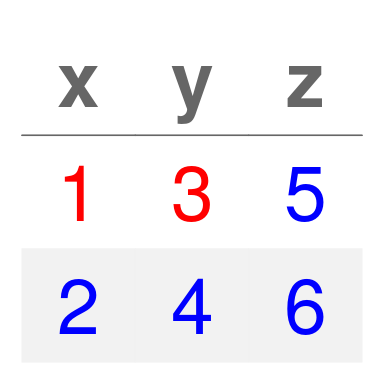• Matrix structures can also be altered (like the column width proportions).

df %>%
grob_matrix() %>%
alter_at(~ 3, columns = 1) %>%
view_grob()• The user can also utilize conditional row selection to determine which rows out of the selected rows and columns to alter.

df %>%
grob_matrix() %>%
aesthetic = "text_color",
value = "blue",
group = "cells"
) %>%
alter_at(
~ "red",
x > 1
) %>%
alter_at(
~ "steelblue",
y < 4,
aesthetic = "background_color"
) %>%
view_grob()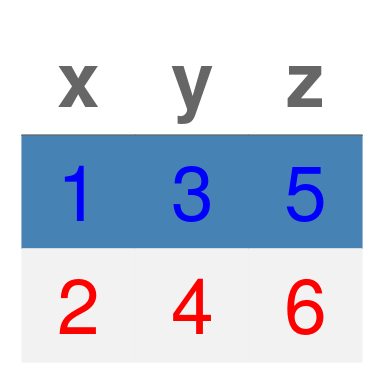• The .f argument is meant to be very flexible, as the user can supply a function to apply to the selected cells to output various aesthetics based on their values.

test_function = function(x) ifelse(x > 3, "purple", "blue")

grob_matrix_with_function = df %>%
grob_matrix() %>%
aesthetic = "text_color",
value = "white",
group = "cells"
) %>%
alter_at(
~ test_function(.),
aesthetic = "background_color"
)

grob_matrix_with_function %>% view_grob()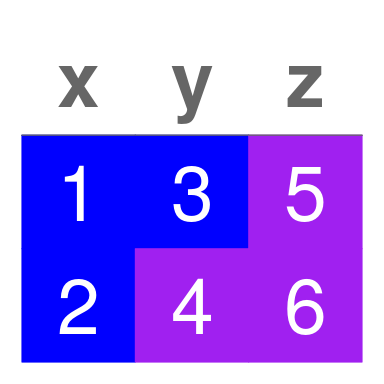• In instances where the user might already have a nicely formatted matrix (where numeric columns might be converted to character columns), the user can supply a separate, underlying matrix/data frame to apply a function to or apply the conditional row selection to.

formatted_df = apply(df, 2, function(x) paste0("F", x))

grob_matrix_with_new_data = formatted_df %>%
grob_matrix() %>%
alter_at(
~ test_function(.),
x > 1,
data = df,
aesthetic = "text_color",
group = "cells"
)

grob_matrix_with_new_data %>% view_grob()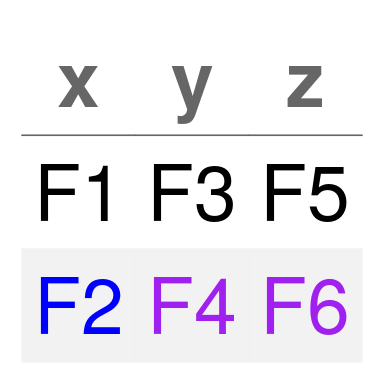## Inserting into Grob Layout

• Once the user has the grob matrices in the place he/she wants them, he/she can simply insert them into grob_layout() inside a grob_col().

gl = grob_layout(
grob_row(grob_col(grob_matrix_with_function)),
grob_row(grob_col(grob_matrix_with_new_data))
)

gl %>% view_grob(height = 100, width = 100)